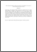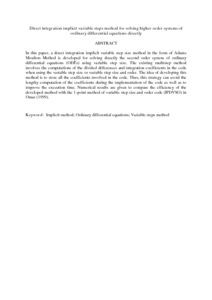# Direct integration implicit variable steps method for solving higher order systems of ordinary differential equations directly

## Citation

Abdul Majid, Zanariah and Suleiman, Mohamed (2006) Direct integration implicit variable steps method for solving higher order systems of ordinary differential equations directly. Sains Malaysiana, 35 (2). pp. 63-68. ISSN 0126-6039

## Abstract

In this paper, a direct integration implicit variable step size method in the form of Adams Moulton Method is developed for solving directly the second order system of ordinary differential equations (ODEs) using variable step size. The existing multistep method involves the computations of the divided differences and integration coefficients in the code when using the variable step size or variable step size and order. The idea of developing this method is to store all the coefficients involved in the code. Thus, this strategy can avoid the lengthy computation of the coefficients during the implementation of the code as well as to improve the execution time. Numerical results are given to compare the efficiency of the developed method with the 1-point method of variable step size and order code (IPDVSO) in Omar (1999).Preview
PDF (Abstract)
Direct integration implicit variable steps method for solving higher order systems of ordinary differential equations directly.pdfView Item